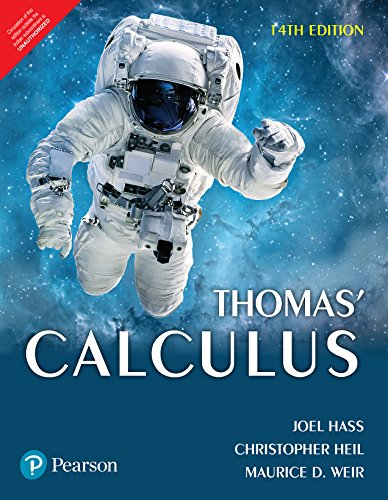# Thomas’ CalculusThomas’ Calculus, Fourteenth Edition, introduces students to the intrinsic beauty of calculus and the power of its applications. For more than half a century, this text has been revered for its clear and precise explanations, thoughtfully chosen examples, superior figures and
time-tested exercise sets.
Features
Strong exercise sets feature a great breadth of problems progressing from skills problems to applied and theoretical problems—to encourage students to think about and practice the concepts until they achieve mastery.
Complete and precise multivariable coverage enhances the connections of multivariable ideas with their single-variable analogues studied earlier in the book.
New to this edition
Updated graphics emphasize clear visualization and mathematical correctness.
New examples and figures have been added throughout all chapters, based on user feedback.
New types of homework exercises, including many geometric in nature, have been added to provide different perspectives and approaches to each topic.
Short URLs have been added to the historical margin notes, allowing students to navigate directly to online information.
New annotations within examples guide the student through the problem solution and emphasize that each step in a mathematical argument is rigorously justified.
Preface
1. Functions
2. Limits and Continuity
3. Derivatives
4. Applications of Derivatives
5. Integrals
6. Applications of Definite Integrals
7. Transcendental Functions
8. Techniques of Integration
9. First-Order Differential Equations
10. Infinite Sequences and Series
11. Parametric Equations and Polar Coordinates
12. Vectors and the Geometry of Space
13. Vector-Valued Functions and Motion in Space
14. Partial Derivatives
15. Multiple Integrals
16. Integrals and Vector Fields
17. Second-Order Differential Equations (Online)
Appendices